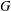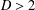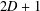Home

# ON THE TOTAL DISTANCE AND DIAMETER OF GRAPHS

## Abstract

The total distance (or Wiener index) of a connected graph$G$ is the sum of all distances between unordered pairs of vertices of$G$ . DeLaViña and Waller [‘Spanning trees with many leaves and average distance’, Electron. J. Combin. 15(1) (2008), R33, 14 pp.] conjectured in 2008 that if$G$ has diameter$D>2$ and order$2D+1$ , then the total distance of$G$ is at most the total distance of the cycle of the same order. In this note, we prove that this conjecture is true for 2-connected graphs.

## References

Hide All
MathJax
MathJax is a JavaScript display engine for mathematics. For more information see http://www.mathjax.org.

# ON THE TOTAL DISTANCE AND DIAMETER OF GRAPHS

## Metrics

### Full text viewsFull text views reflects the number of PDF downloads, PDFs sent to Google Drive, Dropbox and Kindle and HTML full text views.

Total number of HTML views: 0
Total number of PDF views: 0 *Loading metrics...

### Abstract viewsAbstract views reflect the number of visits to the article landing page.

Total abstract views: 0 *Loading metrics...

* Views captured on Cambridge Core between <date>. This data will be updated every 24 hours.

Usage data cannot currently be displayed